### Home > CC2 > Chapter 8 > Lesson 8.3.3 > Problem8-90

8-90.
1. Find the value of each variable in the following figures. Do not use a protractor. Use the properties of angles to help you. Homework Help ✎

•  a.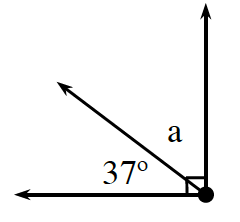b.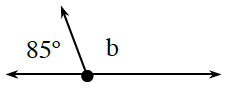c.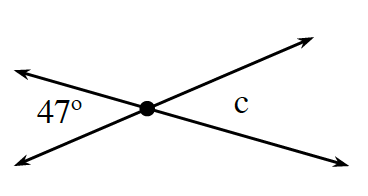d.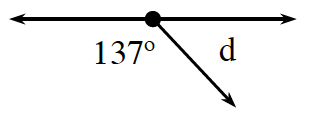e.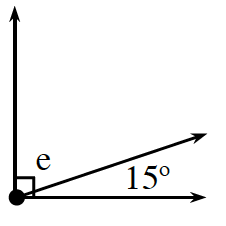f.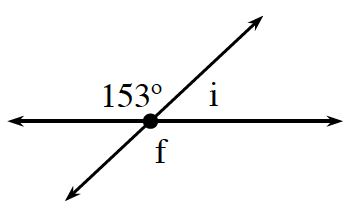g.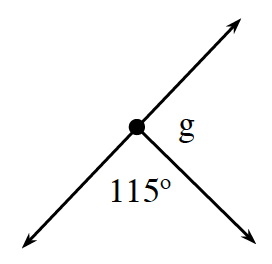h.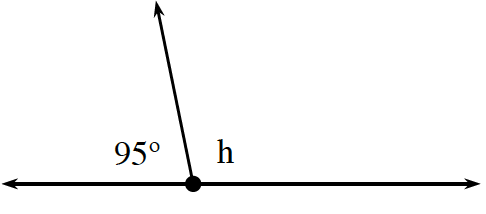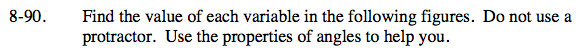37° + a = 90°. Solve for a.

53°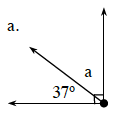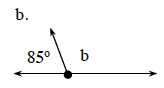These angles are supplementary.
180° − 85° = b

47° and c are vertical angles. What does that tell you about the measure of angle c?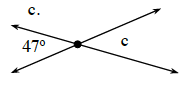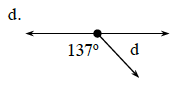Set up an equation relating 137° and d. 137° + d = 180°.

Subtract 137° from both sides.
137° + d = 180°
−137° −137°
d = 43°

43°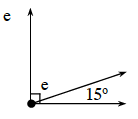e and 15° are complementary angles, meaning their sum is 90°.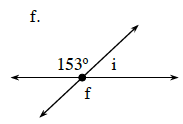f and 153° are vertical angles, and i and 153° are supplementary.

f = 153°, i = 27°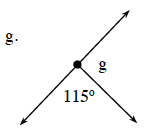115° + g = 180°. Refer to part (d) for the steps to solving this problem.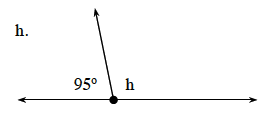95° + h = 180°. Now solve for the value of h.

85°